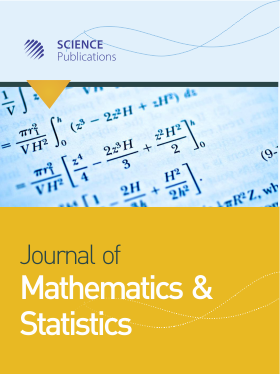Frequency: Continuous
ISSN: 1549-3644 (Print)
ISSN: 1558-6359 (Online)
Research Article Open Access

# Elliptic Weighted Problem with Indefinite Asymptotically Linear Nonlinearity

Hanadi Zahed1 and Laila A. Alnaser1
• 1 Taibah University, Saudi Arabia

## Abstract

The objective of this paper is the study the following nonlinear elliptic problem involving a weight function:

-div(a(x)∇υ) = f(x, u) in Ω and u = 0 on ∂Ω (P)

where, Ω is a regular bounded subset  and ℝN ≥ 2, a(x) is a nonnegative function and f(x, t) is allowed to be sign-changing. We employ variational techniques to prove the existence of a nontrivial solution for the problem (P), under some suitable assumptions, when the nonlinearity is asymptotically linear. Then, we prove by the same method the existence of positive solution when the function f is superlinear and subcritical at infinity.

Journal of Mathematics and Statistics
Volume 17 No. 1, 2021, 13-21

DOI:

Submitted On: 18 October 2020 Published On: 8 February 2021

How to Cite: Zahed, H. & Alnaser, L. A. (2021). Elliptic Weighted Problem with Indefinite Asymptotically Linear Nonlinearity. Journal of Mathematics and Statistics, 17(1), 13-21. https://doi.org/10.3844/jmssp.2021.13.21

• 714 Views